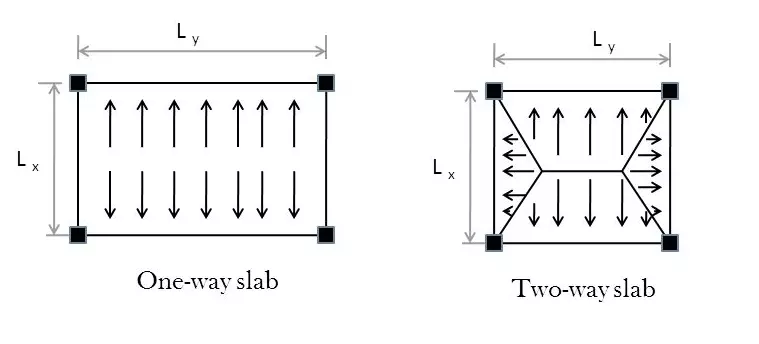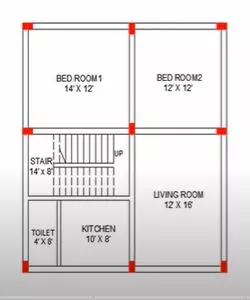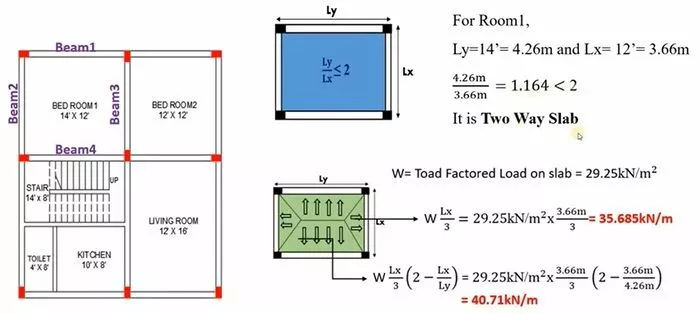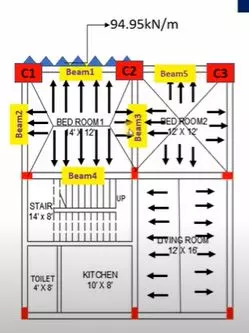# Structural Load Calculation | Load Calculation on Beam, Colum, Slab, Wall and Staircase | Size Calculation for Footing or Foundation:

In a frame-type structure, we must know about the various loads that may be coming on the columns, beams, or slabs. calculate load on beam column wall slab and staircase, structural calculation
The frame structure transfers the load from the slab to beam, from beam to column, and finally to the foundation of the building. structural load calculation

## Definition of Load in Construction:

The stress on a beam, joint, slab, column, etc. The load is the most important consideration when designing structural elements.

When loads are applied to structures, they undergo deformations and displacements, which can cause stresses.

## What is Beam?

The Beam is a horizontal structural member in building construction that carries shear force, bending moment, and transfers loads to columns on both ends of it.

The bottom of the beam experiences tension and the top experiences compression. Therefore, more steel is used at the bottom than at the top. structural load calculation

## What is Column?

A column is a vertical structural member that supports loads from above. For example, a column might support the weight of a ceiling, floor or roof slab or support the weight of a beam.

It is a Compression member, We use columns to hold up buildings and other structures. They can be made of steel, reinforced concrete, wood, or composite materials. The column’s cross-section can take any shape that’s necessary for the load it must sustain.

## What is Slab?

A slab is a flat horizontal surface, such as the floor of a building, that is supported by beams and columns. A slab is usually several inches thick and can be created by pouring concrete over other materials.

Concrete slabs can be poured on-site or off-site or maybe poured in-situ using formwork, but they are typically poured on-site. If reinforcement is required, the concrete can be pre-stressed or rebar can be placed within the formwork before the concrete is poured.

### Load Calculation Procedure on Slab:

When you are calculating load on slab, you have to first check whether it is a one way slab or two way slab structural load calculation

How to check weather’s its one way or two way :

If the ratio of longer span to shorter span is greater than 2 then the slab is considered as one way and if its less than 2 then this slab will be two way slab.

or in simple terms, structural load calculation

L (length) / B (breath) > 2 , then its a One Way Slab and

L / B < 2 then its a Two Way Slab. calculate load on beam

The picture attached below shows the load distribution pattern in one way slab and two way slab. structural load calculationFor one way slab : The formula for load distribution is (Lx/2) x W, where Lx is the shorter span and W is the load (self weight of beam + weight of the wall above the beam + live load on slab). calculate load on beam

Whereas for two way slab, the load distribution pattern follows 2 trapezoid and 2 triangles, so the formula splits into

Triangle region : [(Lx * W)/3] calculate load on beam

Trapezoidal region : [ ((Lx * W)/6) (3 – (Lx/Ly))^2]

The dead load and live load on the structure acts on the slab are efficiently transferred from slab to beams, which in turn transmit the loads to columns. The columns then transmit the loads to the supports and finally to the underlying earth through the foundation. calculate load on beam calculate load on staircase

### Types of Load Acts on Building:

There are mainly two categories of load acts on building structure:

## Types of Load on Column Beam Slab and Wall:

1) Column Self Weight X Number of floors

2) Beams Self Weight per running meter

3) A load of walls per running meter

## Structural Load Calculations for Building:

### Specification of Structural Elements:As per the given house plan: calculate load on column

Thickness of Slab = 130 mm (5″)

Size of All Beams = 230mm x 300mm (9″ x 12″)

Size of All Columns = 230mm x 300mm (9″ x 12″)

Size of flooring = We will calculate the size of footing based on load coming on building

Self Weight of Slab = Slab Thickness x Unit weight of RCC

Self weight of Slab = 0.13 m x 25 KN/m3 = 3.25 KN/m2

Floor Finish Load on Slab = usually we take (1 – 1.5 KN/m2)

Partition Wall Load on Slab = usually we take 1 KN/m2

Waterproofing Material Load = usually we take 1 KN/m2

Total Dead Load o Slab = 3.25 + 1.5 + 1 + 1 = 6.75 KN/m2

Live Load on Slab for residential building = 2 – 3 KN/m2

Live Load on Slab = 3.0 KN/m2

Total Load on Slab = 6.75 + 3.0 = 9.75 KN/m2

Total Load on Slabs (All building Slabs) = 9.75 x 2 = 19.5 KN/m2 (where take 2 for G+1 Story) calculate load on column

Consider Factor of Safety = 1.5

Total Factored Load on Slab = 1.5 x 19.5 = 29.25 KN/m2

Thickness of waist Slab = 150 mm

Riser = 150 mm calculate load on staircase

#### For Going:

Self weight of waist Slab = waist slab thickness x unit weight of RCC x [{sqrt(R2) + sqrt(T2)}/T] calculate load on slab

Self weight of waist Slab = 0.15m x 25 KN/m3 x [{sqrt(0.152) + sqrt(0.252)}/0.25]

Self weight of waist Slab = 4.37 KN/m2

Self weight of Steps = R/2 x 25 = 0.15/2 x 25 = 1.875 KN/m2

Floor Finish Load on Staircase = 1.0 KN/m2

Live Load on Staircase = 3.0 KN/m2

Total Load on Going = 4.37 + 1.875 + 1.0 + 3.0 = 10.25 KN/m2

Ultimate Load on Going = 1.5 x 10.25 = 15.36 KN/m2

#### For Landing:

Self weight of landing = Thickness of landing slab x density

Self weight of landing = 0.15 x 25 = 3.75 KN/m2

Floor finish load on staircase = 1.0 KN/m2

Live Load on Staircase = 3.0 KN/m2

Total Load on Landing = 3.75 + 1.0 + 3.0 = 7.75 KN/m2

Ultimate Load on Landing = 7.75 x 1.5 = 11.625 KN/m2

Self weight of Beam = Size of Beam x unit weight of RCC

Self weight of Beam = (0.23 x 0.30)m x 25 KN/m3 x 2 = 3.45 KN/m (Where 2 is taken for G+1 Story) calculate load on slab

Wall Load on Beam = Thickness of Wall x Height x Unit weight of material

Main Wall Load on Beam = 0.23m x 3m x 19 KN/m3 = 13.11 KN/m

Partition Wall Load on Beam = 0.1m x 3m x 19 KN/m3 = 5.7 KN/m

Parapet Wall Load on Beam = 0.2m x 1.2m x 19 KN/m3 = 4.56 KN/m

Wall Plastering Load on Beam = 0.012m x 3 x 2 x 20.4 KN/m3 = 1.468 KN/mTotal Load on Beam 1 = (3.45 + 13.11 + 4.56 + 1.468 + 40.71) KN/m

Total Load on Beam 1 = 63.29 KN/m calculate load on wall

Total Factored load on Beam 1 = 1.5 x 63.29 = 94.95 KN/mTotal Load on Beam 3 = (3.45 + 13.11 + 1.468 + 35.685 + 35.685) KN/m

Total Load on Beam 3 = 89.39 KN/m calculate load on wall

Total factored Load on Beam 3 = 1.5 x 89.39 = 134.09 KN/m

Total factored load on Beam 2 and 5 = 1.5 x 58.26 = 87.40 KN/m

Self weight of column = size of column x height x unit weight

Self weight of column = (0.23 x 0.3)m x 3m x 25 KN/m3 x 2 = 10.35 KN (where 2 is taken for G+1 Story) calculate load on slab

Total factored load on Beam 1 = 94.95 KN/m

Total factored load on Beam 2 = 87.40 KN/m

Load from Beam = (94.95 x 4.26/2) + (87.40 x 3.66/2) = 362.18 KN

Load on Column 1 = 10.35 KN + 362.18 KN = 372.53 KN

Load from Beam = Half Load from (Beam 1 + Beam 5 + Beam 3)

Load from Beam = (94.95 x 4.26/2) + (87.40 x 3.66/2) + (134.09 x 3.66/2) = 607.57 KN

Load on Column 2 = 10.35 KN + 607.57 KN = 617.92 KN

### Size Calculation for Footing:

#### Size of Footing for Column 1:

Assume, SBC of Soil as 200 KN/m2

Load from Column 1 = 372.53/1.5 = 248.35 KN

Self weight of footing = 10% of unfactored column load

Self weight of footing = 10/100 x 248.35 = 24.83 KN

Total Load on Footing 1 = 248.35 + 24.83 = 273.2 KN

Area of footing = Total Load/SBC of Soil = 273.2/200 = 1.36 m2

If for square footing, we take length = breadth

size of footing L = B = sqrt(1.36) = 1.16m

If for rectangular footing,

Assume one side of footing (B) = 1.0 m Length of footing (L) = 1.36/1.0 = 1.36 = 1.4m (say) calculate load on staircase

Size of footing (L x B) = 1.4 m x 1.0 m

#### Size of Footing for Column 2:

Take SBC of Soil as 200 KN/m2

Load from column 2 = 617.92/1.5 = 411.95 KN

Self weight of footing = 10% of 411.95 = 41.19 KN

Total load on footing 2 = 411.95 + 41.19 = 453.14 KN

Area of footing = Total Load/SBC of Soil = 453.14/200 = 2.26 m2

If for rectangular footing,

Assume one side of footing (B) = 1.4 m

Length of footing = 2.26/1.3 = 1.60 = 1.6m

Size of footing 2 (L x B) = 1.6m x 1.4m

### Note:

To find the size of footing, load considered should be service load for isolated footing.

For any building to find the size of footing, use a combination of loads i,e

1.0 (DL + LL) – Most critical combination is selected

1.5 (DL + LL) – Most critical combination is selected

In above example, we took 1.5 as factor of safety calculate load on staircase

5. ### Difference between Plinth Level, Ground Level, Sill level and Lintel Level

6. Bar Bending Schedule for One Way Slab

## FAQs:

### How is load transferred from slab to beams?

A one-way slab transfers loads in only one direction, whereas a two-way slab transfers loads in two directions.

### What are the Main types of Loads on structures?

For a concrete member, the dead load is calculated by multiplying the volume of the concrete element by its unit weight.

### How is the live load on slab calculated?

The live load on a slab depends on the purpose of the structure, as determined by ASCE standard (ASCE/SEI 10-7). For instance, use 2.4 KN/m2 (50 psf) for offices.

### How do you calculate beam load?

The total load of a beam depends on whether it is made of concrete or steel. The percentage of weight in the material is 2% for both steel and concrete. Hence the Total Weight of the beam = Weight of Concrete + Weight of Steel. The Approximate load of beam having size 230mm x 450mm is around 3.5 KN/m.

Columns are vertical components in a building structure, which are designed to carry the compressive and buckling load. The strength of any column mainly depends on its shape and size of cross-section, length, location and position in the building. The length of a column is generally three times its least lateral cross-sectional dimension.

### What is a Factor of Safety?

A factor of safety is a measure of how much the load-carrying capacity of a system exceeds the actual load.

For a 6″ thick wall with 3.5 meter height and 1.5 meter in length, the load can be calculated per running meter equal to 0.150 x 1.5 x 3.5 x 2000 = 1575 kg which is equivalent to 16 kN/meter. The load per running meter can be calculated for any brick type by following this method.

### What is Wall?

Walls divide spaces (rooms) and provide shelter by keeping the elements out. Walls are generally divided into two categories: Outer walls, which provide safety and shelter, and Inner walls, which separate rooms.

1.Narrotam

Please confirm trapezoidal formula above formula different below different

2.James Adobu

Structural load calculation send me the information

• Thanks for the comments please visit more articles by clicking on “All Posts” tab of the Menu Bar

3.Luate Godfrey

I love the details of the calculation

• Thanks for the comments please visit more articles by clicking on “All Posts” tab of the Menu Bar

4.fekadu debela

Looks great 👍

• Thanks for the comments please visit more articles by clicking on “All Posts” tab of the Menu Bar

5.Santosh Kandel

Thank you for the shearing knowledge !!

• Thanks for the comments please visit more articles by clicking on “All Posts” tab of the Menu Bar

6.Engineer Makakah Silas Odhiambo

Good work must be recommended on this Civil Engineering jobs

• Thanks for the comments please visit more articles by clicking on “All Posts” tab of the Menu Bar

7.Marcellinus Boroh

How load does it work in a wall on slab. In Ghana, I have seen some contractors building on the slab and I thought it not right and what does that mean? What do we do?

• Thanks for the comments please visit more articles by clicking on “All Posts” tab of the Menu Bar

8.Duck

• Thanks for the comments please visit more articles by clicking on “All Posts” tab of the Menu Bar

9.Arun Kumar Gupta

Thanks for good knowledge in civil engineering

• Thanks for the comments please visit more articles by clicking on “All Posts” tab of the Menu Bar

10.Abdishakur

Thanks for sharing knowledge and experience.

• Thanks for the comments please visit more articles by clicking on “All Posts” tab of the Menu Bar

11.Fariha

Thankyou so much for this indepth knowledge!!!you made so many thing easy for me!!!

• Thanks for the comments please visit more articles by clicking on “All Posts” tab of the Menu Bar

12.Innocent

That’s useful information for Civil Engineers

• Thanks for the comments please visit more articles by clicking on “All Posts” tab of the Menu Bar